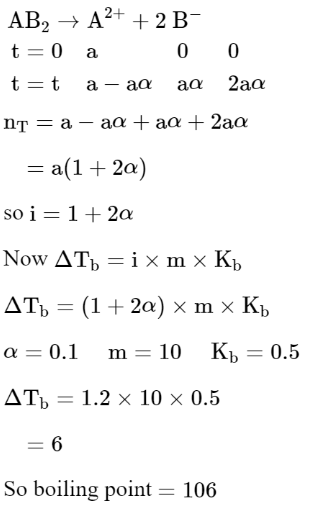# Solve the followingQuestion:

$\mathrm{AB}_{2}$ is $10 \%$ dissociated in water to $\mathrm{A}^{2+}$ and $\mathrm{B}^{-}$. The boiling point of a $10.0$ molal aqueous solution of $\mathrm{AB}_{2}$ is______________ . ${ }^{\circ} \mathrm{C}$. (Round off to the Nearest Integer). [Given : Molal elevation constant of water $\mathrm{K}_{\mathrm{b}}=0.5 \mathrm{~K} \mathrm{~kg} \mathrm{~mol}^{-1}$ boiling point of pure water $\left.=100^{\circ} \mathrm{C}\right]$

Solution:

(106)